# Visualizing CNN Models Through Gradient Weighted Class Activation Mappings

Convolutional Neural Networks(CNNs) and other deep learning networks have enabled extraordinary breakthroughs in computer vision tasks from image classification to object detection, semantic segmentation, image captioning, and many more. While these networks provide superior results, their lack of intuitiveness and understandability makes them hard to interpret. Consequently, a deep learning model is treated as a black box. Often, there is no reasonable idea of where the network is looking in the input image, Which series of neurons activate in the forward-pass and, How the network arrived at its final output.

To move towards the successful integration of deep learning models in our daily lives, it is essential to build trust in them and make them more transparent. This goal of model transparency can be achieved by explaining why the models predict what they predict. The purpose of transparency and explanations is to identify the failure mode, establish appropriate trust and confidence in users, and most importantly teaching humans how to make better decisions.

Gradient-weighted Class Activation Mapping (Grad-CAM) is a technique for producing visual explanations for decisions from a large class of CNN-based models, making them more transparent. The approach uses the gradients of any target output, flowing into the final convolutional layer to produce a localization map highlighting the important regions in the image for predicting the outcome. Grad- CAM applies to a wide variety of CNN model-families without architectural changes or re-training. Grad-CAM is a method for producing heat maps applied to a neural network after its training is complete and the parameters are fixed. Using Grad-CAM, we can visually validate where our network is looking and verify that it is looking at the correct patterns in the image or not.

Grad-CAM does not require a particular CNN architecture and is a generalization of class activation mapping (CAM), a method that does require using a specific architecture. CAM requires an architecture that applies Global Average Pooling (GAP) to the final Convolutional feature maps, restraining the base network from removing all fully connected layers at the end and including a tensor product that takes as input the GAP layer feature maps and outputs the probability for each class.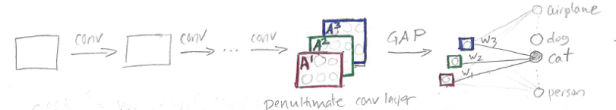Source : glassboxmedicine.com

Grad-CAM’s basic idea is to exploit the spatial information that is preserved through the convolutional layers to understand which parts of an input image were essential for the classification decision. Like CAM, Grad-CAM uses the features obtained from the last convolutional layer of a CNN as they have the best compromise between high-level semantics and detailed spatial information.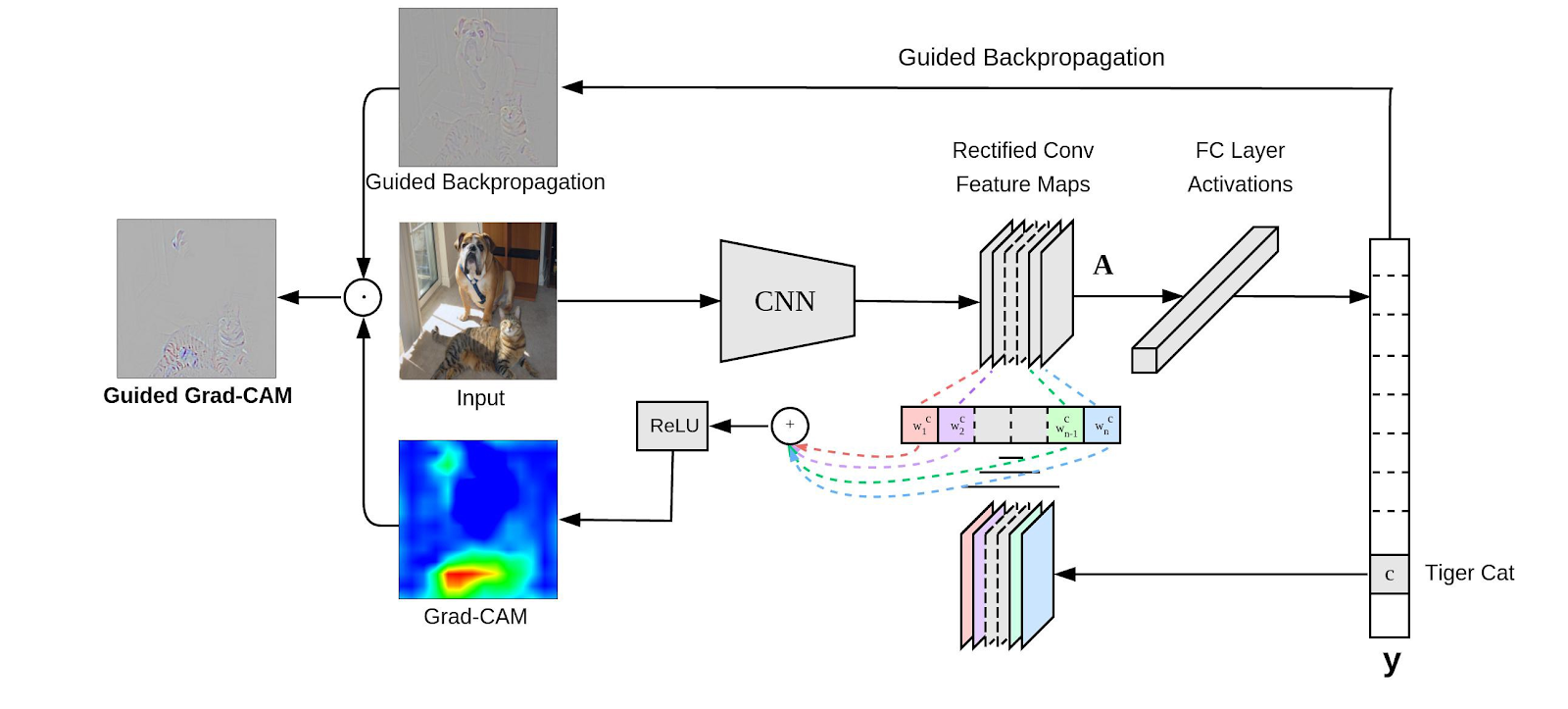source: medium.com

To obtain the class-discriminative localization map, Grad-CAM computes the gradient score for a given class with respect to feature maps of the last convolutional layer. These gradients are global-average-pooled to obtain the importance weights. Similar to CAM, Grad-CAM heat-map is a weighted combination of feature maps followed by a ReLU.ReLU is applied to the linear combination because we are interested in the features that positively influence the class of interest, thus obtaining a precise heat map.

``````import numpy as np
import tensorflow as tf
from tensorflow import keras

# Display
from IPython.display import Image
import matplotlib.pyplot as plt
import matplotlib.cm as cm

model_builder = keras.applications.xception.Xception
img_size = (299, 299)
preprocess_input = keras.applications.xception.preprocess_input
decode_predictions = keras.applications.xception.decode_predictions

last_conv_layer_name = "block14_sepconv2_act"
classifier_layer_names = [
"avg_pool",
"predictions",
]

# The local path to our target image
img_path = keras.utils.get_file(
"african_elephant.jpg", " https://i.imgur.com/Bvro0YD.png"
)

display(Image(img_path))

def get_img_array(img_path, size):
# `img` is a PIL image of size 299x299
# `array` is a float32 Numpy array of shape (299, 299, 3)
array = keras.preprocessing.image.img_to_array(img)
# We add a dimension to transform our array into a "batch"
# of size (1, 299, 299, 3)
array = np.expand_dims(array, axis=0)
return array

img_array, model, last_conv_layer_name, classifier_layer_names
):
# First, we create a model that maps the input image to the activations
# of the last conv layer
last_conv_layer = model.get_layer(last_conv_layer_name)
last_conv_layer_model = keras.Model(model.inputs, last_conv_layer.output)

# Second, we create a model that maps the activations of the last conv
# layer to the final class predictions
classifier_input = keras.Input(shape=last_conv_layer.output.shape[1:])
x = classifier_input
for layer_name in classifier_layer_names:
x = model.get_layer(layer_name)(x)
classifier_model = keras.Model(classifier_input, x)

# Then, we compute the gradient of the top predicted class for our input image
# with respect to the activations of the last conv layer
# Compute activations of the last conv layer and make the tape watch it
last_conv_layer_output = last_conv_layer_model(img_array)
tape.watch(last_conv_layer_output)
# Compute class predictions
preds = classifier_model(last_conv_layer_output)
top_pred_index = tf.argmax(preds)
top_class_channel = preds[:, top_pred_index]

# This is the gradient of the top predicted class with regard to
# the output feature map of the last conv layer

# This is a vector where each entry is the mean intensity of the gradient
# over a specific feature map channel

# We multiply each channel in the feature map array
# by "how important this channel is" with regard to the top predicted class
last_conv_layer_output = last_conv_layer_output.numpy()

# The channel-wise mean of the resulting feature map
# is our heatmap of class activation
heatmap = np.mean(last_conv_layer_output, axis=-1)

# For visualization purpose, we will also normalize the heatmap between 0 & 1
heatmap = np.maximum(heatmap, 0) / np.max(heatmap)
return heatmap

# Prepare image
img_array = preprocess_input(get_img_array(img_path, size=img_size))

# Make model
model = model_builder(weights="imagenet")

# Print what the top predicted class is
preds = model.predict(img_array)
print("Predicted:", decode_predictions(preds, top=1))

# Generate class activation heatmap
img_array, model, last_conv_layer_name, classifier_layer_names
)

# Display heatmap
plt.matshow(heatmap)
plt.show()

# We load the original image
img = keras.preprocessing.image.img_to_array(img)

# We rescale heatmap to a range 0-255
heatmap = np.uint8(255 * heatmap)

# We use jet colormap to colorize heatmap
jet = cm.get_cmap("jet")

# We use RGB values of the colormap
jet_colors = jet(np.arange(256))[:, :3]
jet_heatmap = jet_colors[heatmap]

# We create an image with RGB colorized heatmap
jet_heatmap = keras.preprocessing.image.array_to_img(jet_heatmap)
jet_heatmap = jet_heatmap.resize((img.shape, img.shape))
jet_heatmap = keras.preprocessing.image.img_to_array(jet_heatmap)

# Superimpose the heatmap on original image
superimposed_img = jet_heatmap * 0.4 + img
superimposed_img = keras.preprocessing.image.array_to_img(superimposed_img)

# Save the superimposed image
save_path = "elephant_cam.jpg"
superimposed_img.save(save_path)

display(Image(save_path))``````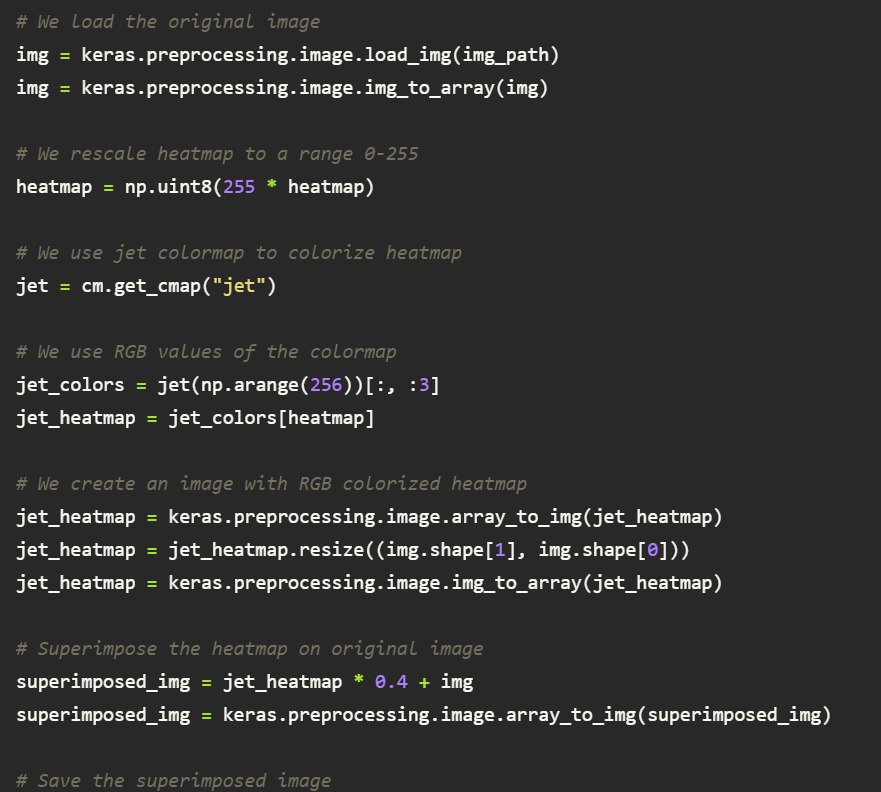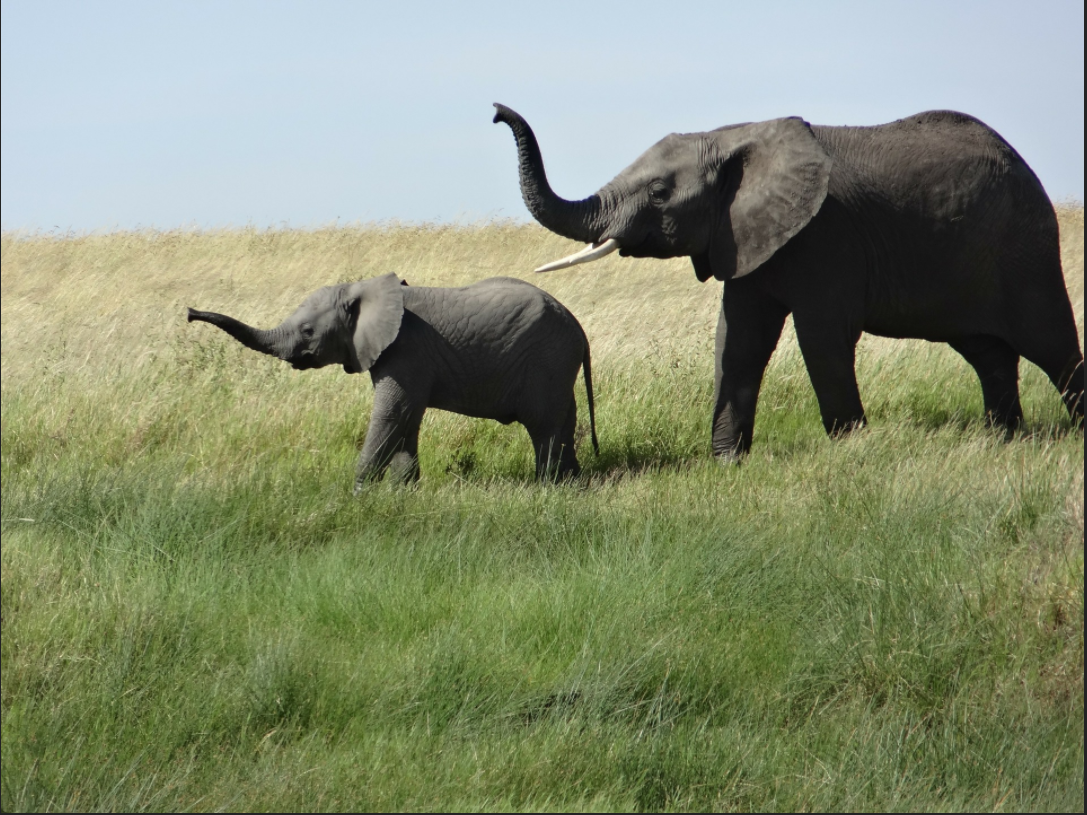Fig: The original image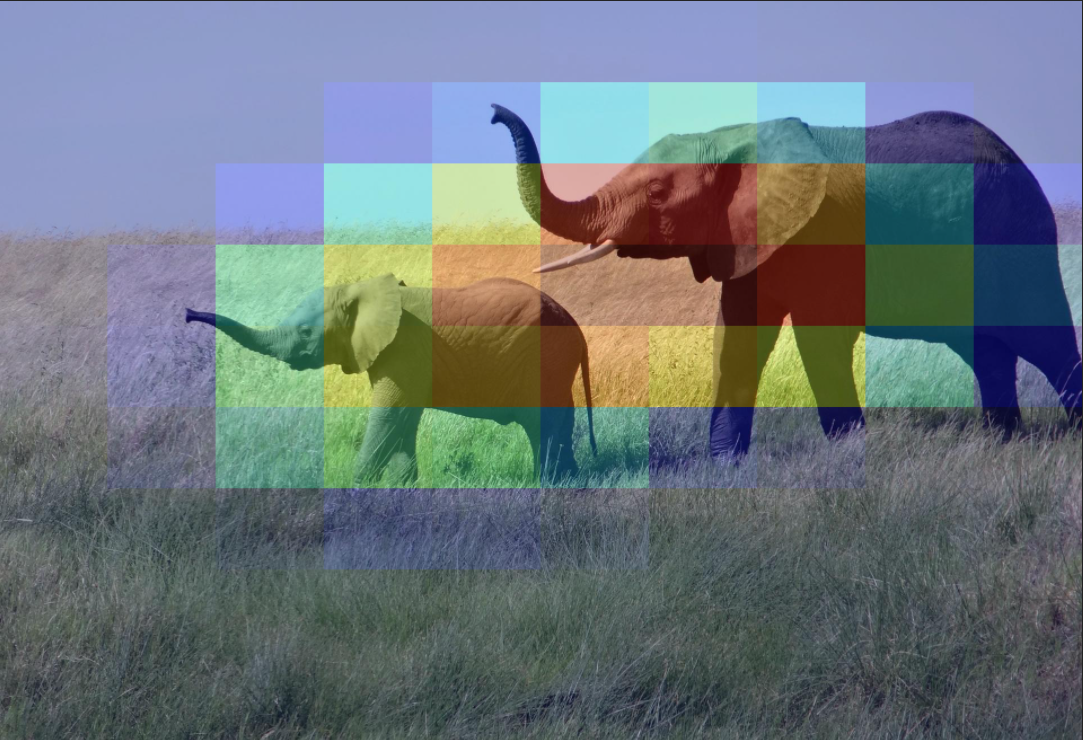Fig: Grad-CAM highlighting the key features of the image